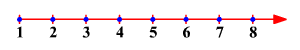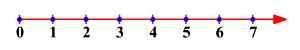# The Natural Numbers

The natural numbers are the numbers that we use to count. The set of natural numbers is usually denoted by the symbol $N$ .

$N$ $=\left\{1,2,3,4,5,6,...\right\}$

The natural numbers are often represented as equally spaced points on a number line, as shown in the figure, increasing forever in the direction of the arrow.The sum or product of two natural numbers is also a natural number. For example,

Sum: $2+3=5$

Product: $\left(2\right)\left(3\right)=6$

This is not always true with differences or quotients of natural numbers. For example, $5-2=3$ is a natural number, but $3-5$ is not. That is, when we subtract a larger natural number from a smaller natural number, we do not get a natural number.

Similarly, $6÷3=2$ is a natural number but $3÷6$ is not. When we divide natural numbers that do not divide evenly, we do not get a natural number.

The set of natural numbers and zero is called the whole numbers . The set of whole numbers is usually denoted by the symbol $W$ .

$W$ $=\left\{0,1,2,3,4,5,6,...\right\}$

The whole numbers are often represented as equally spaced points on a number line , as shown in the figure, increasing forever in the direction of the arrowThe sum or product of two whole numbers is also a whole number, but the difference or quotient of two whole numbers is not always a whole number.